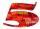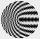# Amazing number

An amazing number is name for such even number, the decomposition product of prime numbers has exactly three not necessarily different factors and the sum of all its divisors is equal to twice that number. Find all amazing numbers.

Result

n =  28

#### Solution:Leave us a comment of example and its solution (i.e. if it is still somewhat unclear...):

Showing 0 comments:Be the first to comment!## Next similar examples:

1. Unknown numberUnknown number is divisible by exactly three different primes. When we compare these primes in ascending order, the following applies: • Difference first and second prime number is half the difference between the third and second prime numbers. • The produ
2. CombinationsHow many different combinations of two-digit number divisible by 4 arises from the digits 3, 5 and 7?
3. NumbersWrite smallest three-digit number, which in division 5 and 7 gives the rest 2.
4. Chebyshev formulaTo estimate the number of primes less than x is used Chebyshev formula: ? Estimate the number of primes less than 30300537.
5. DivisorsThe sum of all divisors unknown odd number is 2112. Determine sum of all divisors of number which is twice of unknown numbers.
6. Theorem proveWe want to prove the sentence: If the natural number n is divisible by six, then n is divisible by three. From what assumption we started?
7. ChordsHow many 4-tones chords (chord = at the same time sounding different tones) is possible to play within 7 tones?
8. PIN - codesHow many five-digit PIN - code can we create using the even numbers?
9. LegsCancer has 5 pairs of legs. The insect has 6 legs. 60 animals have a total of 500 legs. How much more are cancers than insects?
10. LineIt is true that the lines that do not intersect are parallel?
11. SequenceWrite the first 6 members of these sequence: a1 = 5 a2 = 7 an+2 = an+1 +2 an
12. AP - simpleDetermine the first nine elements of sequence if a10 = -1 and d = 4
13. Sequence 2Write the first 5 members of an arithmetic sequence a11=-14, d=-1
14. Three unknownsSolve the system of linear equations with three unknowns: A + B + C = 14 B - A - C = 4 2A - B + C = 0
15. TrigonometryIs true equality? ?
16. SequenceWrite the first 7 members of an arithmetic sequence: a1=-3, d=6.
17. Elimination methodSolve system of linear equations by elimination method: 5/2x + 3/5y= 4/15 1/2x + 2/5y= 2/15